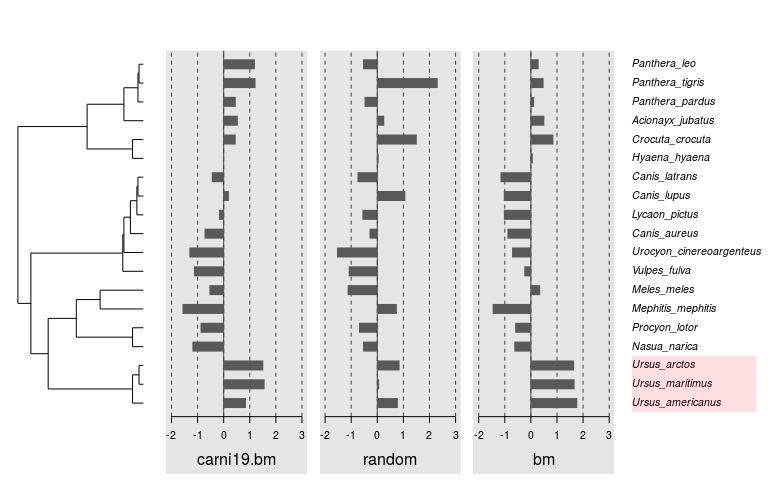# Plotting functions

#### 2020-02-03

The phylosignal package comes with functions designed to plot trait values and phylogeny together. These functions are generics for phylo4d objects. This document present how to use them.

## Data

First, we load the package phylosignal and some others. We will also use the dataset carnivora from adephylo.

library(ape)
library(phylobase)
library(phylosignal)
data(carni19)

Here is a phylogenetic tree of 19 carnivora species.

tre <- read.tree(text = carni19$tre) And we create a dataframe of 3 traits for the 19 carnivora species. - Body mass - Random values - Simulated values under a Brownian Motion model along the tree dat <- data.frame(carni19$bm)
dat$random <- rnorm(dim(dat), sd = 10) dat$bm <- rTraitCont(tre)

We can combine phylogeny and traits into a phylo4d object.

p4d <- phylo4d(tre, dat)

## Basics

Once we have a phylo4d object, we can plot it… There are three plotting functions: barplot, dotplot and gridplot. These functions are actually wrappers of the function multiplot.phylo4d.

barplot(p4d)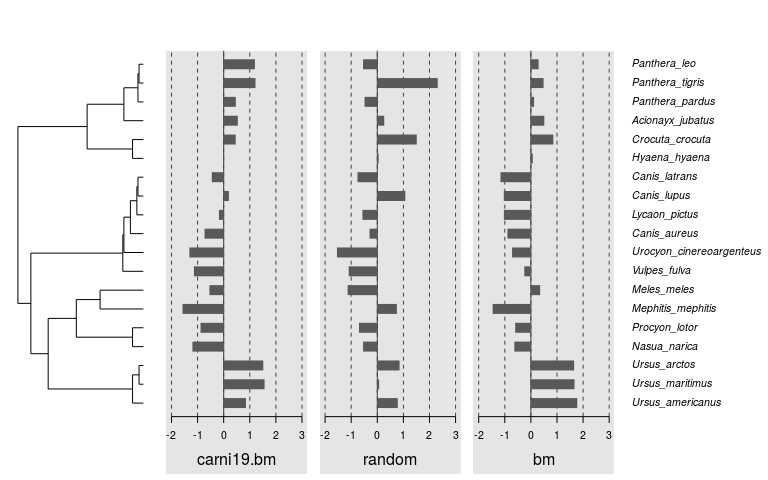dotplot(p4d)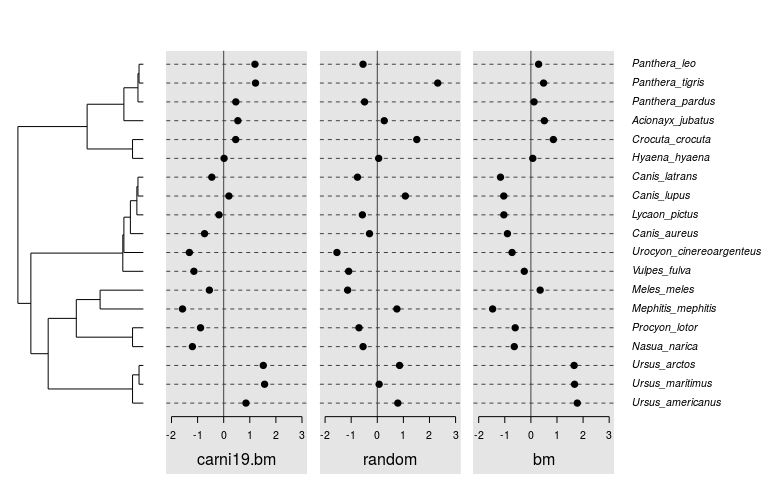gridplot(p4d)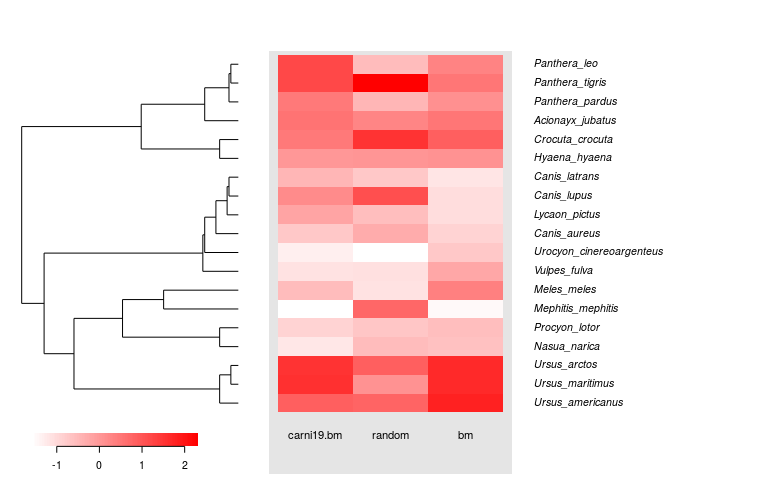Each of these functions can be used with one of the 3 tree styles: phylogram, cladogram and fan. For example, here is a dotplot with a cladogram.

dotplot(p4d, tree.type = "cladogram")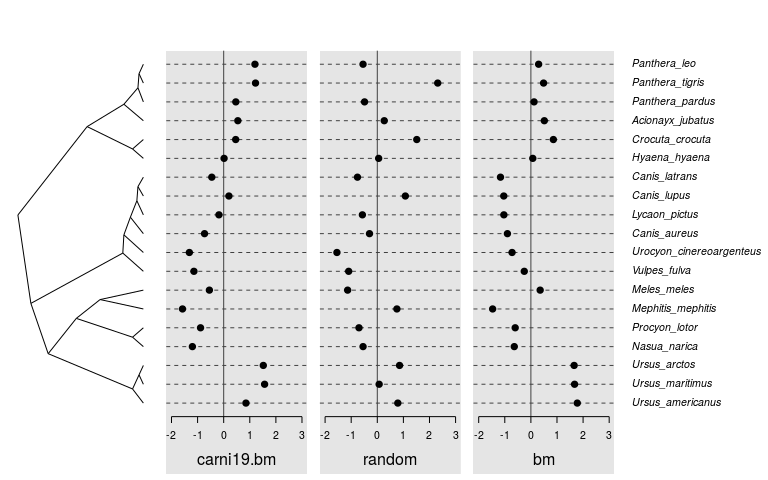And here a gridplot with a fan tree.

gridplot(p4d, tree.type = "fan", tip.cex = 0.6, show.trait = FALSE)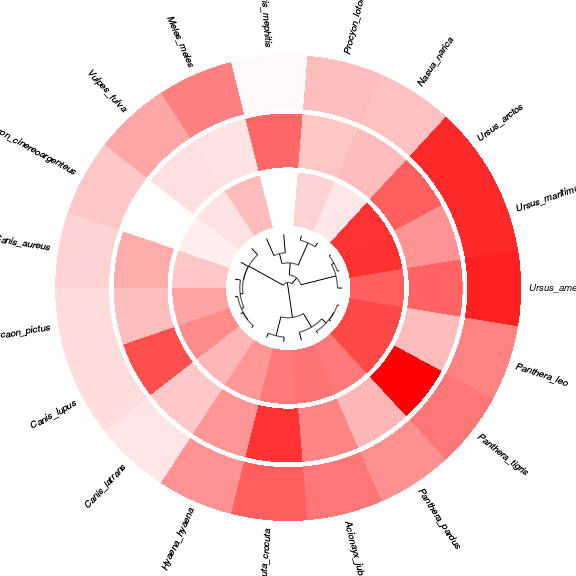Select the ratio of the plot occupied by the tree.

barplot(p4d, tree.ratio = 0.5)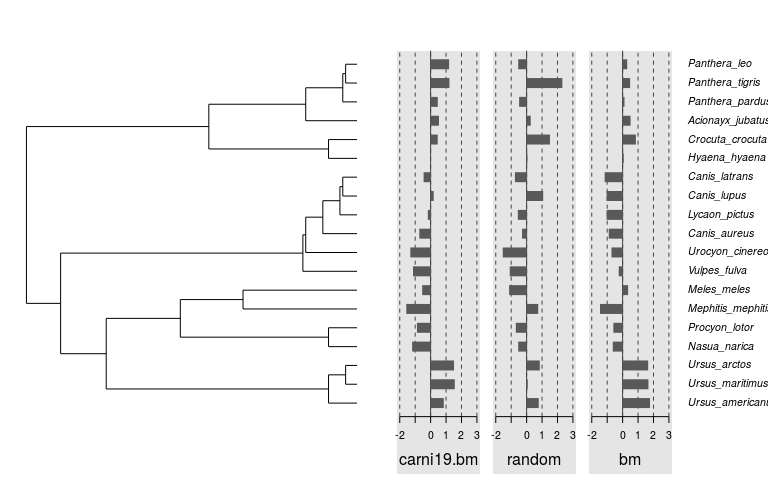Control which traits to plot and their order.

barplot(p4d, trait = c("bm", "carni19.bm"))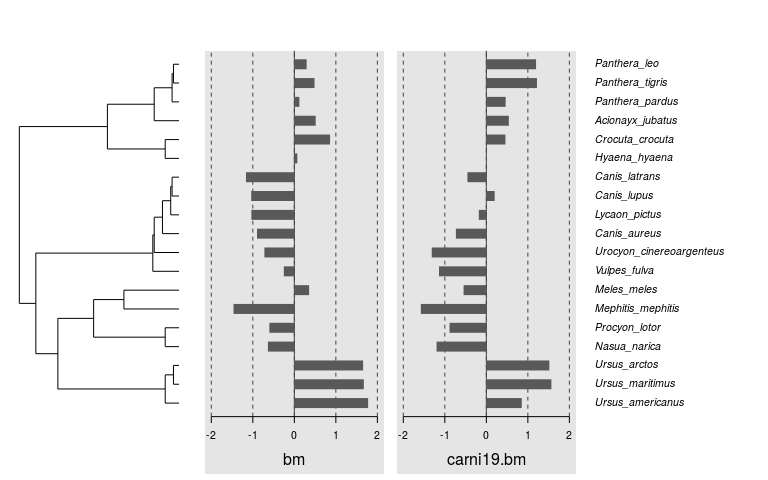mat.e <- matrix(abs(rnorm(19 * 3, 0, 0.5)), ncol = 3,
dimnames = list(tipLabels(p4d), names(tdata(p4d))))
barplot(p4d, error.bar.sup = mat.e, error.bar.inf = mat.e)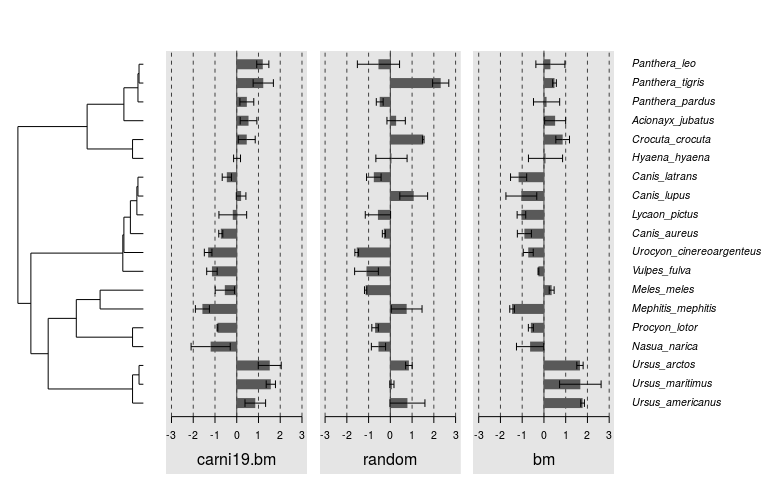It is also possible to open a fan tree with a specified angle.

barplot(p4d, tree.type = "fan", tip.cex = 0.6, tree.open.angle = 160, trait.cex = 0.6)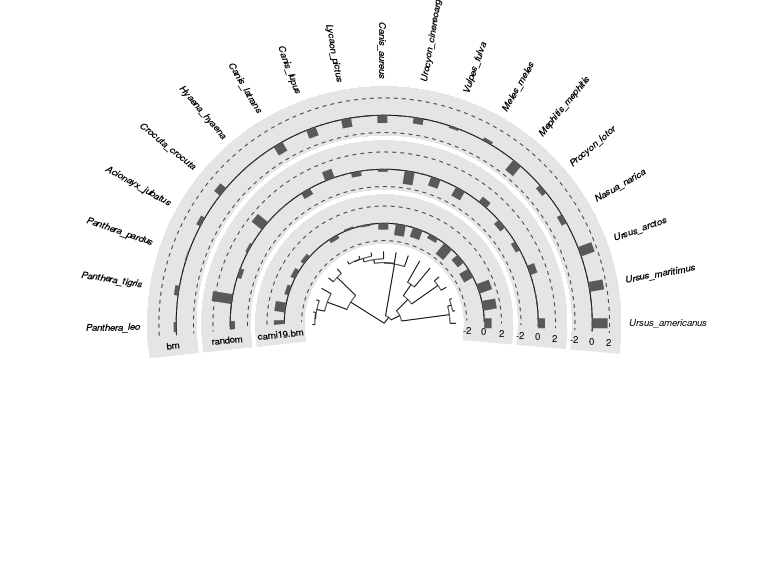## Colors

It’s easy to color bars ‘by species’ with a vector.

barplot(p4d, bar.col = rainbow(19))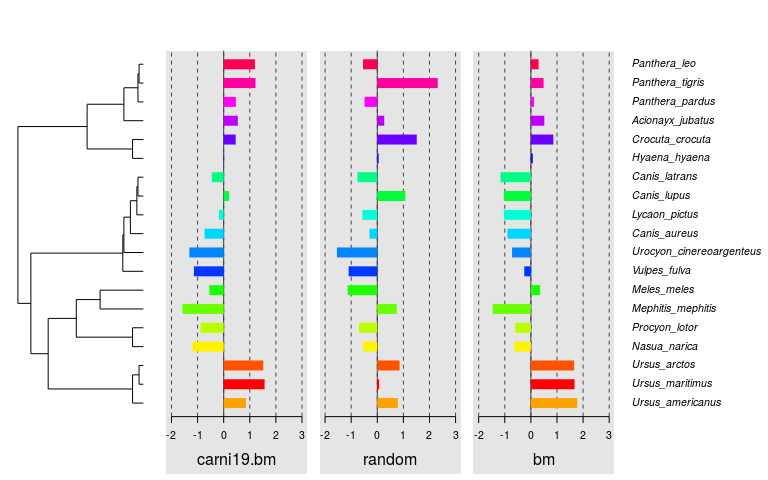And for a finer control, one can use a matrix. Here, negative values in red.

mat.col <- ifelse(tdata(p4d, "tip") < 0, "red", "grey35")
barplot(p4d, center = FALSE, bar.col = mat.col)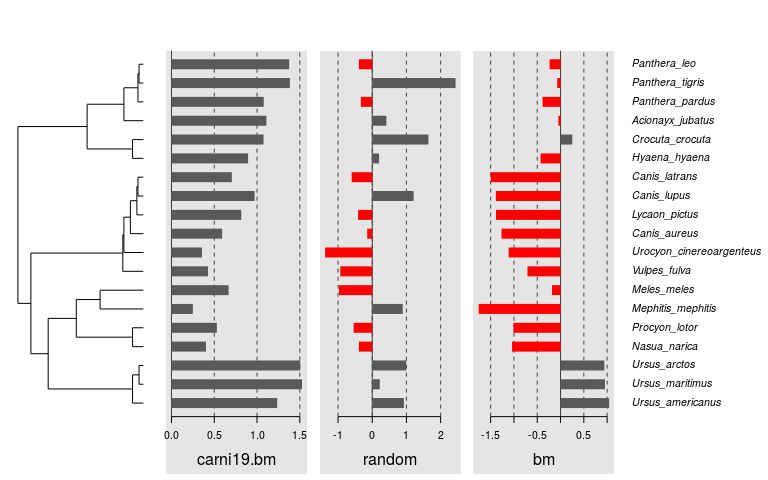Clearly identify traits with colored backgrounds:

barplot(p4d, trait.bg.col = c("#F6CED8", "#CED8F6", "#CEF6CE"), bar.col = "grey35")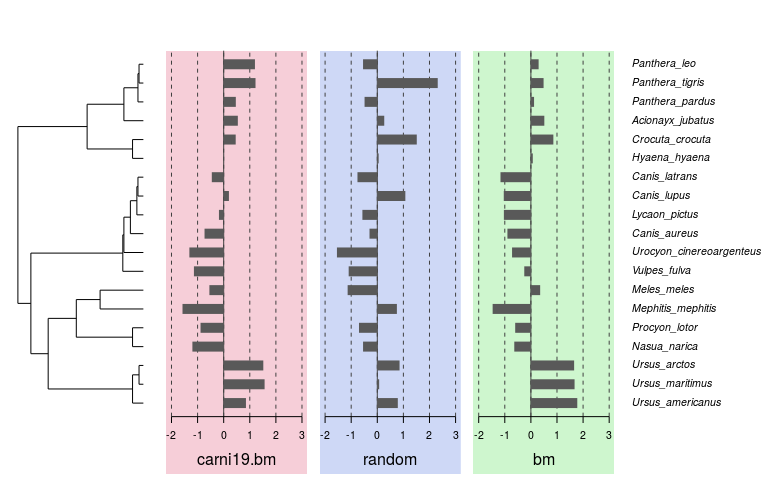For gridplots, cells are colored with a color palette, using the cell.col argument.

gridplot(p4d, tree.type = "fan", tree.ratio = 0.5,
show.trait = FALSE, show.tip = FALSE,
cell.col = terrain.colors(100))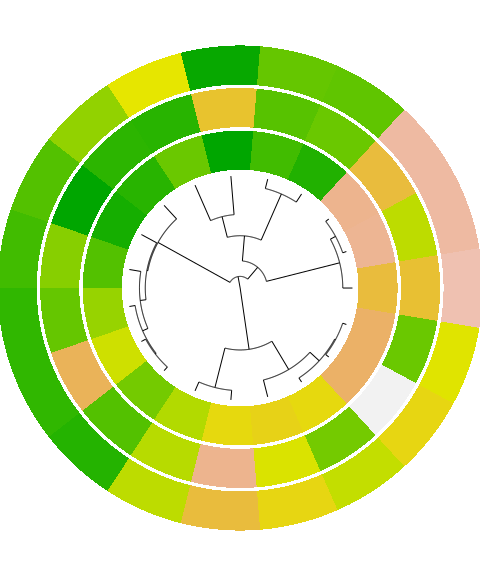Combine arguments for sophisticated plots

tip.col <- rep(1, nTips(p4d))
tip.col[(mat.col[, 2] == "red") | (mat.col[, 3] == "red")] <- 2
barplot(p4d, center = FALSE, trait.bg.col = c("#F6CED8", "#CED8F6", "#CEF6CE"),
bar.col = mat.col, tip.col = tip.col, trait.font = c(1, 2, 2))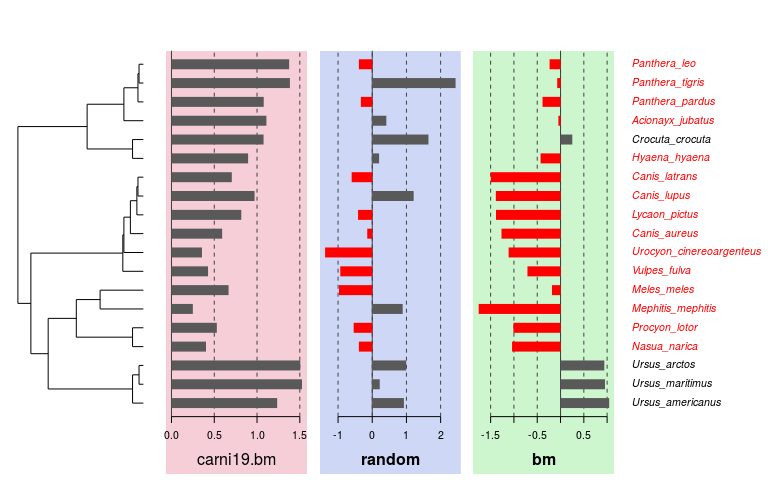You can control many other things. See ?multiplot.phylo4d for more informations.

In R, it is often possible to add graphical elements to a plot after drawing it (eg. with points(), abline(), etc.). This is also possible to use such functions with the plots generated with phylosignal. However, as plots are divided in regions (tree, data, tips), you need special functions to interactively browse among them. These functions are focusTree, focusTraits, focusTips and focusStop. Let’s see how we can use them.

Add a time scale bar to a tree

barplot(p4d)
focusTree()
add.scale.bar()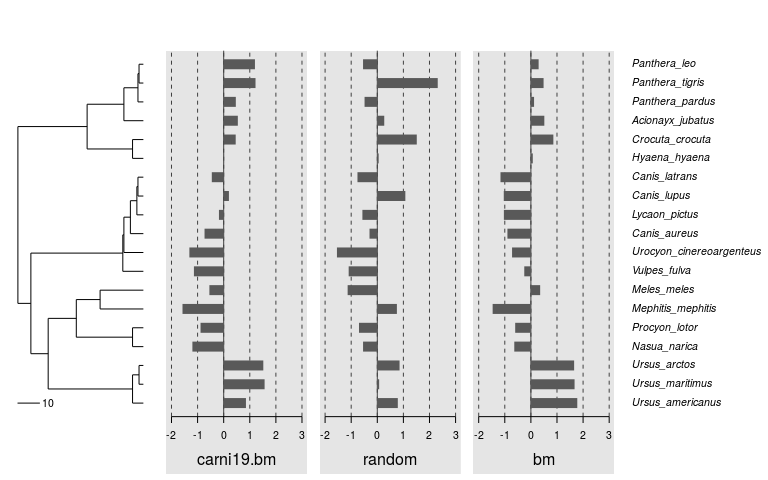Add a vertical red line to the 2nd trait

barplot(p4d)
focusTraits(2)
abline(v = 1, col = 2)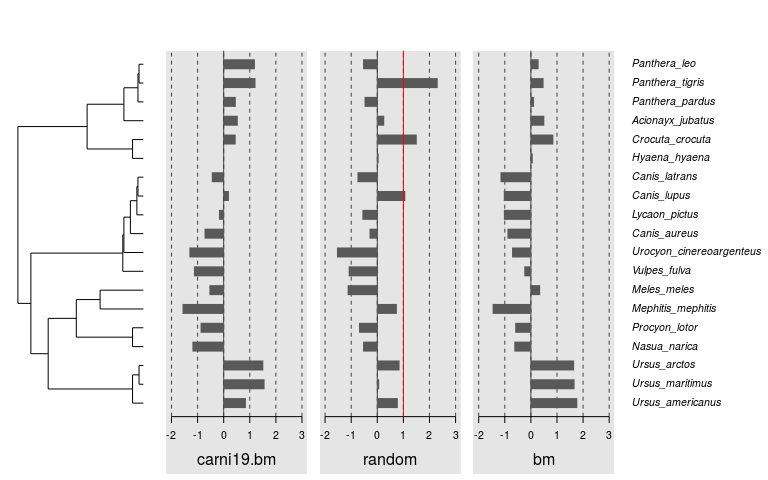Highlight the clade Ursus with a rectangle

barplot(p4d)
focusTips()
rect(xleft = 0, ybottom = 0.5,
xright = 0.95, ytop = 3.5,
col = "#FF000020", border = NA)